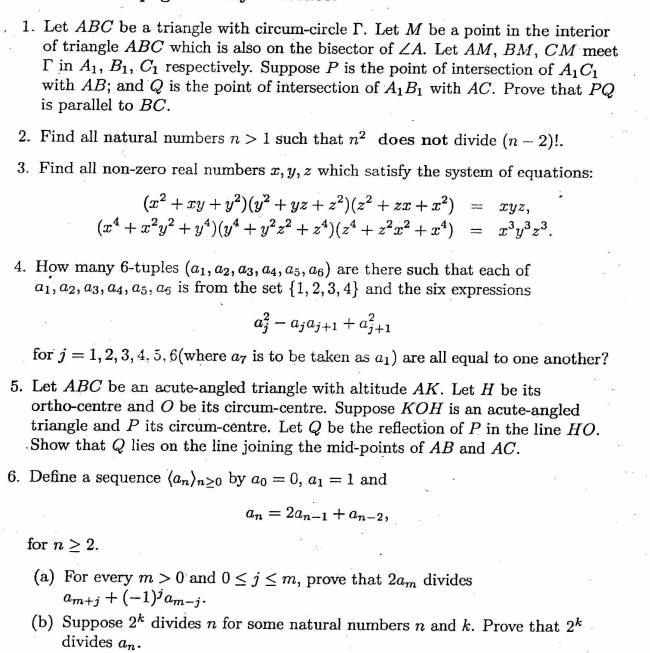# INMO 2010•Dr.House ·

question 3:

(solved by prophet sir)

•Devil ·

Pls don't provide the links bfore the qsns are solved here.

•Dr.House ·

question 6:

(solved by prophet sir)

question 1:

•Devil ·

Number 3 is very easy.

Dividng the given equations give x2y2z2=(x2-xy+y2)(y2-yz+z2)(z2-zx+x2).

Equality is confirmed by A.M-G.M.

•Hari Shankar ·

provided you know something about the signs of x,y,z

•Devil ·

Very similar to the inequality u posted here a few days back.
Basically only possiblity is that only 2 reals can be negative.
So best way is again to proceed by modulus.

•Devil ·

Can anyone confirm my ans to the 2nd one?

Primes and nos. of the form 2p, where p is a prime.

•Hari Shankar ·

•jishrockz dey ·

can anyone tell me the ans of 4? i got 16 tuples.........

n i got the same eq in 3 as soumik replied........
bt i used the identity (a3+b3) and (a3-b3)

•Dr.House ·

this is hwere u can get all the questions and the answers..

hit on the question number to get the solution.

•jishrockz dey ·

where the hell?? i can't find the solution of 4........

•jishrockz dey ·

in 4, i got the condition that a1=a3=a5 and a2=a4=a6........

then tuples were of form p,q,p,q,p,q where p,q are from {1,2,3,4} ........

•Dr.House ·

its been answered a little later:

see this q4:

•albert ·

I got the answer of second question as all primes, twice the primes, 8 and 9.
Did someone else get the same?

•Shubhodip ·

5)

Easy to see that PQ bisects OH

Its wellknown that the midpoint of OH is the ninepoint center N of triangle ABC

Let the midpoints of the segments AB,AC,BH,CH be P,T,S,R respectively.

Then PTRS is a rectangle. Since RS is the perpendicular OK , the circumcenter P of triangle OKH lies on the line RS.

Since N is the nine point center of ABC, a half turn about N maps S to T and R to P. So N is the center of the rectangle PTRS. Since P lies in RS, and NP= NQ, we obviously have that Q lies on PT and we are done.

•